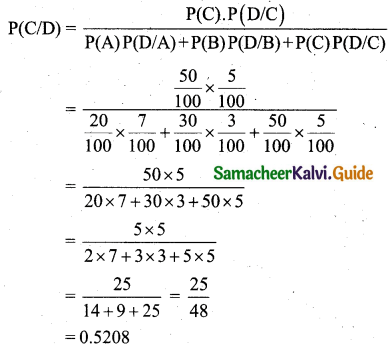Tamilnadu State Board New Syllabus Samcheer Kalvi 11th Business Maths Guide Pdf Chapter 8 Descriptive Statistics and Probability Ex 8.2 Text Book Back Questions and Answers, Notes.

## Tamilnadu Samacheer Kalvi 11th Business Maths Solutions Chapter 8 Descriptive Statistics and Probability Ex 8.2

### Samacheer Kalvi 11th Business Maths Descriptive Statistics and Probability Ex 8.2 Text Book Back Questions and Answers

Question 1.
A family has two children. What is the probability that both the children are girls given that at least one of them is a girl?
Solution:
Let B denote a boy and G denote a girl.
Then the sample, S = {BG, GB, BB, GG}.
∴ n(S) = 4
Let E be the event that both children are girls.
Let F be the event that atleast one of them is a girl.
Then E = {GG}, n(E) = 1
F = {BG, GB, GG}, n(F) = 3
P(F) = $$\frac{n(\mathrm{F})}{n(\mathrm{S})}=\frac{3}{4}$$
E ∩ F = {GG}, n(E ∩ F) = 1
P(E ∩ F) = $$\frac{n(\mathrm{E} \cap \mathrm{F})}{n(s)}=\frac{1}{4}$$
Required Probability P(F/E) = $$\frac{P(E \cap F)}{P(F)}=\frac{\frac{1}{4}}{\frac{3}{4}}=\frac{1}{3}$$Question 2.
A die is thrown twice and the sum of the number appearing is observed to be 6. What is the conditional probability that the number 4 has appeared at least once?
Solution:
When a die is thrown twice, the sample is S = {(1, 1), (1, 2), (1, 3), (1, 4), (1, 5), (1, 6)
(2, 1), (2, 2), (2, 3), (2, 4), (2, 5), (2, 6)
(3, 1), (3, 2), (3, 3), (3, 4), (3, 5), (3, 6)
(4, 1), (4, 2), (4, 3), (4, 4), (4, 5), (4, 6)
(5, 1), (5, 2), (5, 3), (5, 4), (5, 5), (5, 6)
(6, 1), (6, 2), (6, 3), (6, 4), (6, 5), (6, 6)}
n(S) = 36
Let A be the event of getting the sum of the numbers is 6.
Let B be the event of the number 4 has appeared atleast once.
We have to find P(B/A)
A = {(1, 5), (5, 1), (2, 4), (4, 2), (3, 3)}, n(A) = 5
∴ P(A) = $$\frac{5}{36}$$
B = {(1, 4), (2, 4), (3, 4), (4, 4), (5, 4), (6, 4), (4, 1),(4, 2), (4, 3), (4, 5), (4, 6)}
A ∩ B = {(2, 4), (4, 2)}, n(A ∩ B) = 2
P(A ∩ B) = $$\frac{2}{36}$$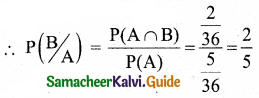Question 3.
An unbiased die is thrown twice. Let the event A be an odd number on the first throw and B the event odd number on the second throw. Check whether A and B events are independent.
Solution:
When a die is thrown twice, the sample space is S = {(1, 1), (1, 2), (1, 3), (1, 4), (1, 5), (1, 6)
(2, 1), (2, 2), (2, 3), (2, 4), (2, 5), (2, 6)
(3, 1), (3, 2), (3, 3), (3, 4), (3, 5), (3, 6)
(4, 1), (4, 2), (4, 3), (4, 4), (4, 5), (4, 6)
(5, 1), (5, 2), (5, 3), (5, 4), (5, 5), (5, 6)
(6, 1), (6,2), (6, 3), (6, 4), (6, 5), (6, 6)}
n(S) = 36
The event A is odd number on the first throw
∴ A = {(1, 1), (1, 2), (1, 3), (1, 4), (1, 5), (1, 6)
(3, 1), (3, 2), (3, 3), (3, 4), (3, 5), (3, 6)
(5, 1), (5, 2), (5, 3), (5, 4), (5, 5), (5, 6)}
n(A) = 18
P(A) = $$\frac{18}{36}=\frac{1}{2}$$
The event B is odd number on the second throw.
B = {(1, 1), (1, 3), (1, 5), (2, 1), (2, 3), (2, 5)
(3, 1), (3, 3), (3, 5), (4, 1), (4, 3), (4, 5)
(5, 1), (5, 3), (5, 5), (6, 1), (6, 3), (6, 5)}
n(B) = 18
P(B) = $$\frac{18}{36}=\frac{1}{2}$$
A ∩ B = {(1, 1), (1, 3), (1, 5)
(3, 1), (3, 3), (3, 5)
(5, 1), (5, 3), (5, 5)}
n(A ∩ B) = 9
P(A ∩ B) = $$\frac{9}{36}=\frac{1}{4}$$
Also P(A) . P(B) = $$\frac{1}{2} \cdot \frac{1}{2}=\frac{1}{4}$$
Thus P(A ∩ B) = P(A) . P(B)
∴ A and B are independent events.Question 4.
Probability of solving specific problem independently by A and B are $$\frac{1}{2}$$ and $$\frac{1}{3}$$ respectively. If both try to solve the problem independently, find the probability that the problem is (i) solved, (ii) exactly one of them solves the problem.
Solution:
Given P(A) = $$\frac{1}{2}$$ and P(B) = $$\frac{1}{3}$$
(i) The probability that problem is solved = The probability that atleast one solving the problem
= 1 – P(none of them solving the problem)
= 1 – $$P(\bar{A} \cap \bar{B})$$
= 1 – $$\mathrm{P}(\overline{\mathrm{A}}) \cdot \mathrm{P}(\overline{\mathrm{B}})$$
= 1 – (1 – $$\frac{1}{2}$$) (1 – $$\frac{1}{3}$$)
= 1 – ($$\frac{1}{2}$$) ($$\frac{2}{3}$$)
= 1 – $$\frac{1}{3}$$
= $$\frac{2}{3}$$
(ii) P(exactly one of them solves the problem)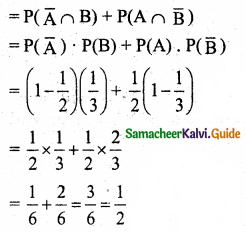Question 5.
Suppose one person is selected at random from a group of 100 persons are given in the following:What is the probability that the man selected is a Psychologist?
Solution:
Total number of men = 50
Number of men psychologist = 15
The probability of selecting a psychologist given that a man has been already selected is $$\frac{15}{50}=\frac{3}{10}$$Question 6.
Two urns contain the set of balls as given in the following table.One ball is drawn from each urn and find the probability that (i) both balls are red, (ii) both balls are of the same colour.
Solution:
(i) Let A be the event of drawing a red from urn 1,
P(A) = $$\frac{6}{25}$$
Let B be the event of selecting a red ball in urn 2.
P(B) = $$\frac{7}{25}$$
∴ P(both balls are red) = P(A) . P(B) [∵ the events are independent]
= $$\frac{6}{25} \times \frac{7}{25}$$
= $$\frac{42}{625}$$
Let W1, R1, B1 represents white, red, and black balls drawn from urn 1 and W2, R2, B2 represents white, red, and black balls from urn 2.
P(both balls are of the same colour) = P(W1W2) + P(R1R2) + P(B1B2) [∵ Events are mutually exclusive]
= P(W1) P(W2) + P(R1) P(R2) + P(B1) P(B2) [∵ Events are independent]
= $$\frac{10}{25} \times \frac{3}{25}+\frac{6}{25} \times \frac{7}{25}+\frac{9}{25} \times \frac{15}{25}$$
= $$\frac{1}{625}$$ [30 + 42 + 135]
= $$\frac{207}{625}$$

Question 7.
The bag I contain 3 Red and 4 Black balls while another Bag II contains 5 Red and 6 Black balls. One ball is drawn at random from one of the bags and it is found to be red. Find the probability that it was drawn from Bag I.
Solution:Let E1 be the event of choosing the first bag, E2 be the event of choosing the second bag.
Let A be the event of drawing a red ball.
Then P(E1) = $$\frac{1}{2}$$, P(E2) = $$\frac{1}{2}$$
Also P(A/E1) = P(Drawing a red ball from bag I) = $$\frac{3}{7}$$
P(A/E2) = P(Drawing a red ball from bag II) = $$\frac{5}{11}$$
The probability of drawing a ball from bag I, being given that it is red, is P(E1/A)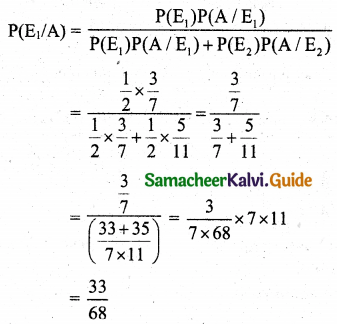Question 8.
The first of three urns contains 7 White and 10 Black balls, the second contains 5 White and 12 Black balls, and the third “contains 17 White balls and no Blackball. A person chooses an urn at random and draws a ball from it. And the ball is found to be White. Find the probabilities that the ball comes from (i) the first urn, (ii) the second urn, (iii) the third urn.
Solution:Let E1, E2, and E3 be the event of choosing the first, second, and third urn.
Let A be the event of drawing a white ball.
then P(E1) = P(E2) = P(E3) = $$\frac{1}{3}$$
P(A/E1) = P(drawing a white ball from the first urn) = $$\frac{7}{17}$$
P(A/E2) = P(drawing a white ball from the second urn) = $$\frac{5}{17}$$
P(A/E3) = P(drawing a white ball from the third urn) = $$\frac{17}{17}$$
(i) The probability of drawing a ball from the first urn, being given that it is white, is P(E1/A),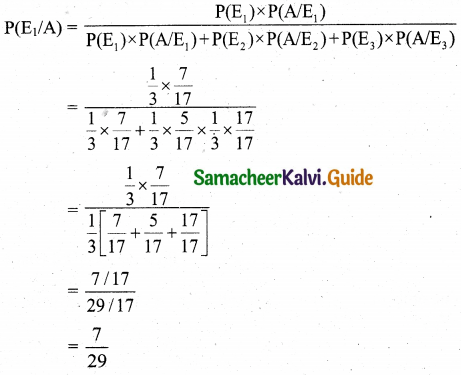(ii) the second urn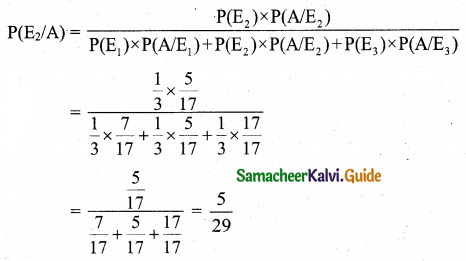(iii) the third urnQuestion 9.
Three boxes B1, B2, B3 contain lamp bulbs some of which are defective. The defective proportions in box B1, box B2 and box B3 are respectively $$\frac{1}{2}$$, $$\frac{1}{3}$$ and $$\frac{1}{4}$$. A box is selected at random and a bulb is drawn from it. If the selected bulb is found to be defective, what is the probability that box B1 was selected?
Solution:
Given that B1, B2 and B3 represent three boxes.
Then P(B1) = P(B2) = P(B3) = $$\frac{1}{3}$$
Let A be the event of selecting a defective bulb.
Then P(A/B1) = P(drawing a defective bulb from B1) = $$\frac{1}{2}$$
P(A/B2) = P(drawing a defective bulb from B2) = $$\frac{1}{8}$$
P(A/B3) = P(drawing a defective bulb from B3) = $$\frac{3}{4}$$
The probability of drawing a defective bulb from B1, being given that it is defective, is P(B1/A).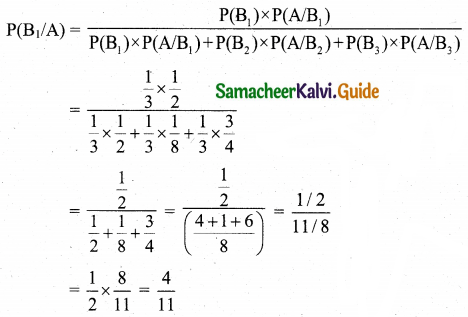Question 10.
Three horses A, B, C are in the race. A is twice as likely to win as B and B is twice as likely to win as C. What are their respective probabilities of winning?
Solution:
Given that A is twice as likely to win as B.
Therefore, A : B = 2 : 1
(or) A : B = 4 : 2 …….(1)
Also given that B is twice as likely to win as C.
Therefore, B : C = 2 : 1 ………(2)
From (1) and (2),
A : B : C = 4 : 2 : 1
∴ A = 4k, B = 2k, C = 1, where C is a constant of proportionality.
Probability of A wining is $$\frac{4 k}{7 k}=\frac{4}{7}$$
Probability of B wining is $$\frac{2 k}{7 k}=\frac{2}{7}$$
Probability of C wining is $$\frac{k}{7 k}=\frac{1}{7}$$

Question 11.
A die is thrown. Find the probability of getting (i) a prime number, (ii) a number greater than or equal to 3.
Solution:
Let S be the sample when a die is thrown.
Then S = {1, 2, 3, 4, 5, 6}, n(S) = 6
Let A be the event of getting a prime number.
A = {2, 3, 5}, n(A) = 3
Let B be the event of getting a number greater than or equal to 3.
B = {3, 4, 5, 6}, n(B) = 4
(i) P(a prime number) = $$\frac{n(\mathrm{A})}{n(\mathrm{S})}=\frac{3}{6}=\frac{1}{2}$$
(ii) P(a number ≥ 3) = $$\frac{n(\mathrm{B})}{n(\mathrm{S})}=\frac{4}{6}=\frac{2}{3}$$Question 12.
Ten cards numbered 1 to 10 are placed in a box, mixed up thoroughly, and then one card is drawn randomly. If it is known that the number on the drawn card is more than 4. What is the probability that it is an even number?
Solution:
Let S = {1, 2, 3,…, 10}, n(S) = 10
Let A be the event of drawing a number greater than 4.
Then A= {5, 6, 7, 8, 9, 10}, n(A) = 6
∴ P(A) = $$\frac{n(\mathrm{A})}{n(\mathrm{S})}=\frac{6}{10}$$
Let B be the event of getting a even number.
Then B = {2, 4, 6, 8, 10}
Now A ∩ B = {6, 8, 10}, n(A ∩ B) = 3
P(A ∩ B) = $$\frac{3}{10}$$
We have to find P (B/A)Question 13.
There are 1000 students in a school out of which 450 are girls. It is known that out of 450, 20% of the girls studying in class XI. A student is randomly selected from 1000 students. What is the probability that the selected student is from class XI given that the selected student is a girl?
Solution:
Number of students = 1000 i.e., n(S) = 1000
Let A be the event of selecting a girl student from class XI.
Let B be the event of selecting a girl student
Number of girls = 450; n(B) = 450
∴ P(B) = $$\frac{n(\mathrm{B})}{n(\mathrm{S})}=\frac{450}{1000}$$
Number df girls studying ih XI is 20% of 450 = $$\frac{20}{100} \times 450$$ = 90
i.e., n(A ∩ B) = 90
∴ P(A ∩ B) = $$\frac{n(\mathrm{A} \cap \mathrm{B})}{n(\mathrm{S})}=\frac{90}{1000}$$
We have to find P(A/B)Question 14.
From a pack of 52 cards, two cards are drawn at random. Find the probability that one is a king and the other is a queen.
Solution:
Number of kings is 4
Number of queens is 4
Two cards are drawn.
Probability of one king and one queen is,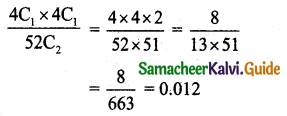Question 15.
A card is drawn from a pack of playing cards and then another card is drawn without the first being replaced. What is the probability of drawing (i) two aces, (ii) two spades?
Solution:
A number of cards 52.
The number of aces 4.
(i) Probability of drawing two aces(ii) The probability of drawing two spadesQuestion 16.
A company has three machines A, B, C which produces 20%, 30%, and 50% of the product respectively. Their respective defective percentages are 7, 3, and 5. From these products, one is chosen and inspected. If it is defective what is the probability that it has been made by machine C?
Solution:
The probability of the product produced by machine A is P(A) = $$\frac{20}{100}$$
The probability of the product produced by machine B is P(B) = $$\frac{30}{100}$$
The probability of the product produced by the machine C is P(C) = $$\frac{50}{100}$$
Let D be the event of selecting a defective product.
Then P(D/A) = The probability of selecting a defective product produced by the machine A = $$\frac{7}{100}$$
P(D/B) = the probability of selecting a defective product produced by the machine B = $$\frac{3}{100}$$
and P(D/C) = The probability of selecting a detective product produced by the machine C = $$\frac{5}{100}$$